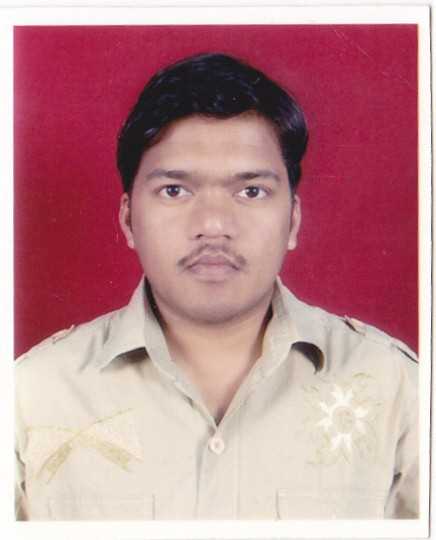# Dynamic Tutorials and Services

Main | cwa4 | Registration | Login

 Wednesday, 01/Dec/2021, 11:13 AM Welcome Guest | RSSQuestion Papers
 Study Materials
 Competitive Exams
 Computer Basic
 Paper 4: Business Mathematics and Statistics Fundamentals (One Paper: 3 hours: 100 marks) 1. Arithmetic 10% Average, mixtures, Ratios and proportions, Computation of interest, Discounting of bills 2. Algebra 15% a)      Real and imaginary number, rational and irrational number, Set theory and simple application of Venn diagram Truth table and its applications, Indices and surds, Variation, Logarithms b)      Permutations and Combinations, Compound interest c)       Linear simultaneous equations (3 variables only), Quadratic equations, Solution of linear inequalities (by geometric method only) 3. Mensuration 15% Area and perimeter of triangles, circles, parallelogram, regular polygon Volume and surface of cube, prism, cylinder, pyramid, cone, and spheres (including zone and segments) 4. Co-ordinate Geometry 10% Plain co-ordinate Geometry ( Rectangular Cartesian co-ordinates only Length of line segments, Section ratio, Gradient of a line, equation of straight line, Circles, parabola, ellipse and hyperbola ( standard forms only) 5.Calculus 15% a)      Constant &Variables, Functions, Limit & Continuity, b)      Differentiability & Differentiation, Derivatives and their use, Successive & partial differentiation, Maxima & Minima, Maxima & Minima under constraint using Lagrange transform c)       Indefinite Integrals: as primitives, integration by substitution, integration by part d)      Definite Integrals: Evaluation of standard integrals, as area under curve Applications of Calculus 6. Statistical Methods 35% a)      Data tabulation and presentation, frequency distribution b)      Measures of Central Tendency (Mean, Median, mode) c)       Measurement of Dispersion (range, mean deviation, standard deviation, variance) Measures of Skewness & kurtosis
Calendar
 «  December 2021  » Su Mo Tu We Th Fr Sa 1 2 3 4 5 6 7 8 9 10 11 12 13 14 15 16 17 18 19 20 21 22 23 24 25 26 27 28 29 30 31
 StatisticsTotal online: 1 Guests: 1 Users: 0

 3rd Floor, H. P. Jallan Building, G. N. B . Road, Tinsukia, Assam - 786125